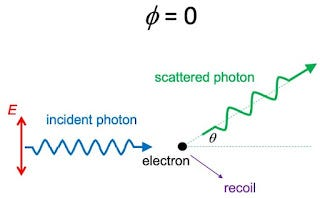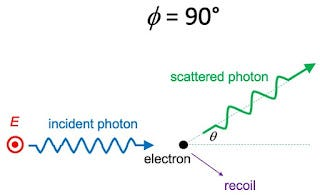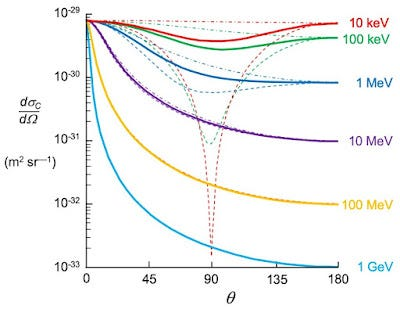# Klein-Nishina Formula for Polarized Photons

## φ = 0

The incident and scattered photon directions define a plane. Assume the electric field associated with the incident photon lies in this plane, as shown in the drawing below. From a classical point of view, the electric field will cause the electron to oscillate, resulting in dipole radiation (a process called Thomson scattering). A dipole radiates perpendicular to its direction of oscillation, but not parallel to it. Therefore, you get scattering for θ = 0 and 180°, but not for θ = 90°.A schematic diagram of Compton scattering for polarized light with φ = 0.

## φ = 90°

For Thomson scattering, if the electric field oscillates perpendicular to the scattering plane (shown below) then all angles θ are perpendicular to the dipole and therefore should radiate equally. This effect is also evident in a quantum analysis unless x >> 1.A schematic diagram of Compton scattering for polarized light with φ = 90°.The differential cross section for Compton scattering. The incident photon energy for each curve is shown on the right. The solid curves are for unpolarized light, the dashed curves are for light with φ = 0, and the dash-dot curves are for φ = 90°. Adapted from Fig. 15.6 in IPMB.

--

--